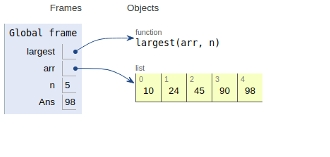# Python Program to find largest element in an array

In this article, we will learn about the solution and approach to solve the given problem statement.

## Problem statement

Given an array as an input, we need to find the largest element in the array.

## Approach

• We initialize max as the first element.
• After this, we traverse the given array from the second element till end.
• For every traversed element, we compare it with the current value of max
• if it is greater than max, then max gets updated.
• Otherwise, the statement surpasses

Let’s see the implementation below −

## Example

Live Demo

def largest(arr,n):
#maximal element
max = arr
for i in range(1, n):
if arr[i] > max:
max = arr[i]
return max
# main
arr = [10, 24, 45, 90, 98]
n = len(arr)
Ans = largest(arr,n)
print ("Largest in the given array is",Ans)

## Output

Largest in the given array is 98

All the variables and functions are declared in global scope as shown in the figure below.